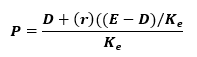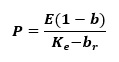# Dividend Policy Assignment Help

Dividend is the amount paid regularly by a company to its shareholders on a regular interval out of its unappropriated profits or reserves. Dividend Policy is the policy which deals with the paying of such dividends. The policy designs the method of paying and the amount of the dividend. The unappropriated profits and the long term earning power of the business influence the decisions for the implementation of a dividend policy.

In case the company has excess cash available with itself it will either give away the dividends to the shareholders or buyback its shares unless and otherwise it is willing to invest amount in projects or looks forward to expanding. In case there is no project available with a positive NPV i.e. with a return which exceeds the normal hurdle rate, then the amount is supposed to be paid to shareholders as the dividend.

Dividend policy has always been a challenge for the management. It is difficult to decide the amount of dividends due to various expectations of different shareholders and on the basis of share price. Thus it becomes really challenging which is why Dividend Policy is also known as Dividend Puzzle.

## Importance of Dividend Policy in Business:

It is said that the companies which pay dividends regularly are regarded as favorable ones whereas those who do not are opposed by the shareholders. The companies which pay regular dividends maintain the interest of the shareholders and those who don’t make the shareholders insecure about their investments.

There are basically two Models for forming of a proper Dividend Policy. These are:

1. Walter’s Model
2. Gordon’s Model.

## Walter’s Model:

### Assumptions of the Walter model:

1. Retained earnings are the only source of financing investments in the firm, there is no external finance involved.
2. The cost of capital, ke and the rate of return on investment, r are constant i.e. even if new investments decisions are taken, the risks of the business remains same.
3. The firm's life is endless i.e. there is no closing down.

### Mathematical Expression:Where,

• P = Market price of the share
• D = Dividend per share
• r = Rate of return on the firm's investments
• ke = Cost of equity
• E = Earnings per share'

## Dividend Policy Assignment Help By Online Tutoring and Guided Sessions from AssignmentHelp.Net

The market price of the share consists of the sum total of:

• the present value of an infinite stream of dividends
• The present value of an infinite stream of returns on investments made from retained earnings.

#### Criticism:

1. The assumption of no external financing apart from retained earnings, for the firm make further investments is not really followed in the real world.
2. The constant r and ke are seldom found in real life, because as and when a firm invests more the business risks change.

### Explanation of the Model:

There are two treatments of the profits in the company; either it is retained in the business and put into further investment or it is paid out to the shareholders in the form of dividends. If dividend is paid to the shareholders, the shareholders try to maximize their gain by reinvesting it in the business. Thus, if the same amount is retained by the business and invested then it is assumed that the business must earn a rate of interest from the investment which must at least be equal to the returns of the company. If r<ke; it means that the returns of the company is better than the rate of return on investment. Hence, the amount must be paid out as dividends. On the other hand if r>ke, the amount must be retained in the business and must be invested in the investments.

In a nutshell:

• If r>ke, the firm should have zero payout and make investments.
• If r< ke, the firm should have 100% payouts and no investment of retained earnings.
• If r=ke, the firm is indifferent between dividends and investments.

## Gordon’s Model:

### The Assumptions of the Gordon model:

Gordon's assumptions are similar to the ones given by Walter. However, there are two additional assumptions proposed by him:

1. The product of retention ratio b and the rate of return r give us the growth rate of the firm g.
2. The cost of capital ke, is not only constant but greater than the growth rate i.e. ke>g.

### Mathematical Expression:Where,

• P = Market price of the share
• E = Earnings per share
• b = Retention ratio (1 - payout ratio)
• r = Rate of return on the firm's investments
• ke = Cost of equity
• br = Growth rate of the firm (g)

### Explanation of Model:

Investors are always opposed to risk situations. They always avoid risk situations this is why they take the prudence concept in consideration. According to the shareholders the capital gains by the selling of the shares is a riskier option as compared to the dividends. The dividends ensure a timely pay whereas the capital gains might be risky with accordance to the future predictions. Thus the shareholders discount the future capital gains to increase the dividend payout in present. This is done by discounting the future capital gains at a higher rate than the firm’s earnings which in return increases the value of the share. Thus it means when the retention ratio is increased by the shareholders; a higher discounting rate is required.

Thus, different approaches can be used to study the dividend policies. Both the models had a logical view point but also had drawbacks.